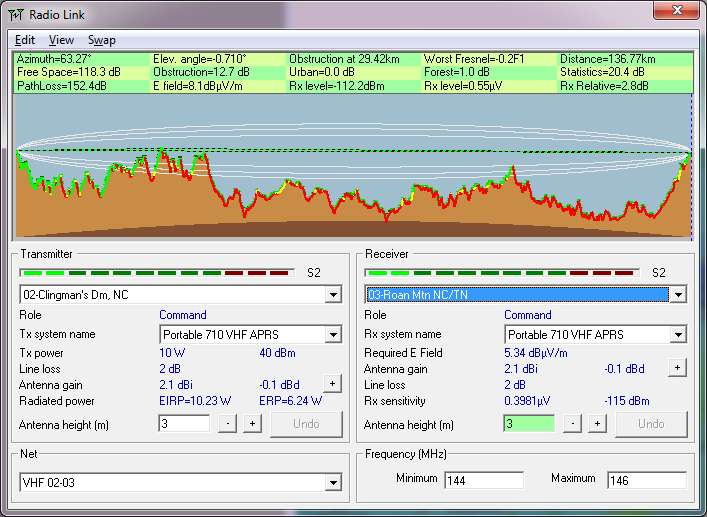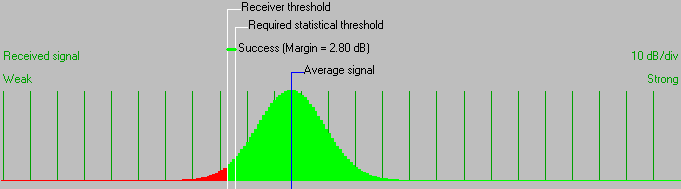• Distance between 02-Clingman's Dm, NC and 03-Roan Mtn NC/TN is 136.8 km (85.0 miles)
• True North Azimuth = 63.27°, Magnetic North Azimuth = 68.93°, Elevation angle = -0.7097°
• Terrain elevation variation is 1466.3 m
• Propagation mode is diffraction, single obstruction, 0.2F1 at 29.4km
• Average frequency is 145.000 MHz
• Free Space = 118.3 dB, Obstruction = 12.7 dB, Urban = 0.0 dB, Forest = 1.0 dB, Statistics = 20.4 dB
• Total propagation loss is 152.4 dB (interference mode (optimistic))
• System gain from 02-Clingman's Dm, NC to 03-Roan Mtn NC/TN is 155.2 dB
• System gain from 03-Roan Mtn NC/TN to 02-Clingman's Dm, NC is 155.2 dB
• Worst reception is 2.8 dB over the required signal to meet 95.000% of situations